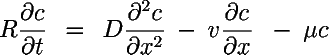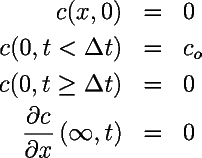# EPA On-line Tools for Site Assessment Calculation

Transport from a Continuing or Pulse Concentration Source

##### One Dimensional Transport Equation

This calculator generates a breakthrough curve* at a receptor and uses a one-dimensional model to determine the

1. First arrival time for a contaminant (above a specified threshold concentration),
2. Maximum Concentration, and
3. Duration of Contaminantion above the specified threshold

*The breakthrough curve is the time history of concentration at a receptor located a specified distance from the contaminant source.See bottom of page for equations solved.

b )

mg/L mg/l

mg/L
C o

##### Advective Travel Time

earlier than the advective travel time

One Dimensional Transport From a Pulse SourceR = retardation factor []
D = dispersion constant [L2/T]
v = seepage velocity [L/T]
μ = first order decay constant [1/T]

with the initial and boundary conditionsretardation factor

R = 1 + ρ b kd

R = retardation factor
ρ b = bulk density = ρ s (1-θ)
ρ s = solids density
θ = porosity
k d = (soil) distribution coefficient = f oc K oc
f oc = fraction organic carbon
K oc = organic carbon/water partition coefficient

travel time t tr = x R /v

t tr = advective travel time *
v = seepage velocity
R = retardation factor
x = distance
* assuming one-dimensional, steady flow, constant gradient

Contact Jim Weaver to ask a technical question on this material.